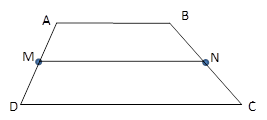Chapter 4.4, Problem 14E### Elementary Geometry for College St...

6th Edition
Daniel C. Alexander + 1 other
ISBN: 9781285195698

#### Solutions

Chapter
Section### Elementary Geometry for College St...

6th Edition
Daniel C. Alexander + 1 other
ISBN: 9781285195698
Textbook Problem
24 views

# In Exercises 11 to 16, the drawing shows trapezoid ABCD with A B ¯ | | D C ¯ ; also, M and N are midpoints of A D ¯ and B C ¯ , respectively.Exercises 11-16Given: A B = 7 x + 5 , D C = 4 x − 2 and M N = 5 x + 3 Find: x .

To determine

To Find:

The value of x.

Explanation

Consider the following trapezoid ABCD.

Given:

AB=7x+5, DC=4x2 and MN=5x+3.

Also in the given trapezoid, AB¯||DC¯.

M is the midpoint of the side AD¯ and N is the midpoint of the side BC¯.

MN is the median of the trapezoid ABCD.

We know that the length of the median (m) of a trapezoid is the average of the lengths of the bases

### Still sussing out bartleby?

Check out a sample textbook solution.

See a sample solution

#### The Solution to Your Study Problems

Bartleby provides explanations to thousands of textbook problems written by our experts, many with advanced degrees!

Get Started

#### Are the following graphs of functions? Explain. a. b. c. d.

Applied Calculus for the Managerial, Life, and Social Sciences: A Brief Approach

#### Find the mean for the following set of scores: 2, 7, 9, 4, 5, 3, 0, 6

Essentials of Statistics for The Behavioral Sciences (MindTap Course List)

#### Evaluate the definite integral. 12e1/xx2dx

Single Variable Calculus: Early Transcendentals, Volume I

#### The solution to y = y2 with y(1)=13 is: a) y=1x+2 b) y=lnx+13 c) y=12x216 d) y=12x16

Study Guide for Stewart's Single Variable Calculus: Early Transcendentals, 8th

#### The vector represented by where A(4, 8) and B(6, 6)is:

Study Guide for Stewart's Multivariable Calculus, 8th